# Presse universelle MecaMotion par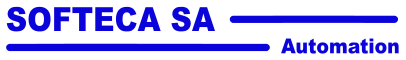Navigation: Part program instructions "Conditional/unconditional jump" instruction

"Conditional/unconditional jump" instruction

The jump instruction is used in conjunction with the label instruction. When executing the jump, the part-program will position itself on the selected label (jump destination).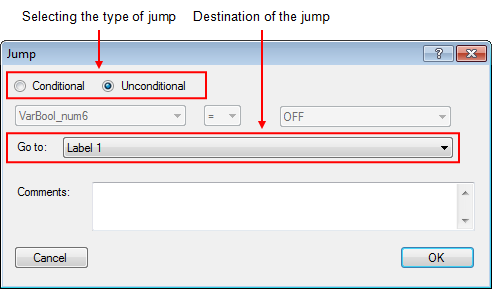Figure 1: Jump programming window

There are two types of jumps:

Unconditional Jump

Conditional Jump

Below is a list of the instruction's input and output parameters:

Parameter name

Declaration

Type of data

Default value

Description

With or without condition

Input

--

Condition

Choice of jump type

Compared variable

Input

BOOL, REAL, LREAL, INT or DINT

--

Compared variable

Type of comparison

Input

--

=

Type of comparison,  "=", ">", "<" ou "<>"

Comparing

Input

BOOL, REAL, LREAL, INT or DINT

--

Comparing variable

Go to

Input

--

--

Destination label

Table 1: List of instruction parameters

Unconditional jump

The unconditional jump is the easiest. As soon as the part-program arrives on this instruction, the program goes directly to the instruction that follows the destination label.

Conditional jump

The conditional jump allows you to add a condition to perform the jump. If the condition is not met, the jump is not performed and the following instruction is executed.

You can compare variables of type BOOL (0 or 1) or compare variables of type REAL, LREAL, INT or DINT with values.

Compare if a Boolean variable is "=" or "<>" of "0" or "1".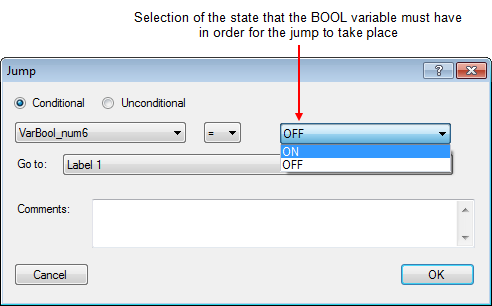Figure 2: Comparison of a BOOL variable with a constant

Compare whether a variable of type REAL, LREAL, INT or DINT is "<" ">" "=" or "<>" in relation to a constant/variable.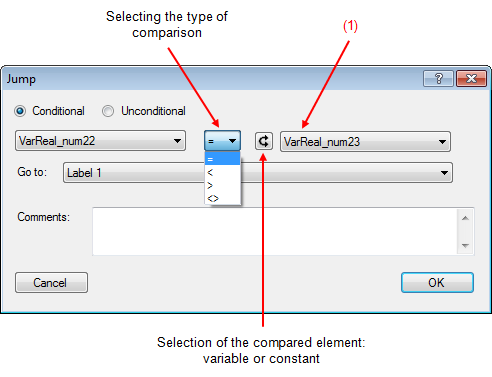Figure 3: Comparison of a REAL variable with a REAL variable

(1) You can compare variables of different types.

For example:

Compare that the value of a REAL variable  is greater than the value of a INT variable.

Compare that the value of a DINT variable is equal to the value of a REAL variable.

...

 Example of use: We want to activate a Boolean variable when the maximum force measured during a positioning is greater than or equal to 45[N]. In this example, the jump instruction allows the maximum force measured during positioning to be compared with the setpoint force. If the maximum force is greater than or equal to the setpoint force, we set the Boolean variable "B_force_ok" to "1" and, if the maximum force is less than the setpoint force, the variable "B_force_ok" remains at "0", meaning that the force is not sufficient.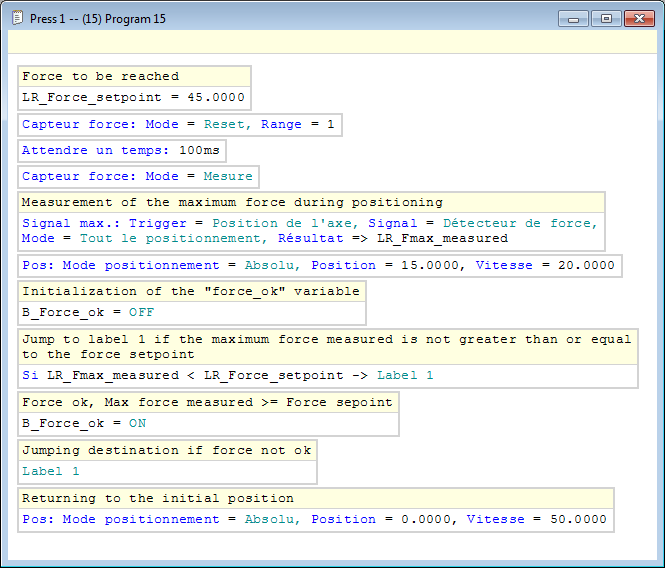Figure 4: Example of using the jump instruction